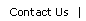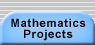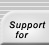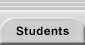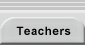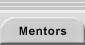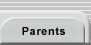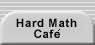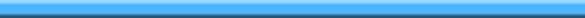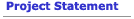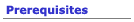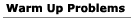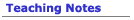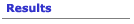## Warm Ups for the Trains Problem

Imagine a number system that only uses 1s and 2s. It has numbers in it like: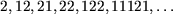• List all the three digit numbers in this system. Explain how you can be sure that you haven’t missed any and that you haven’t listed any twice.
• Write a number-listing-rule for this system. Test it out on 4-digit numbers. If it works, it should produce the full list of 4 digit numbers in the system.
• Use your algorithm to decide how many n-digit numbers use just the digits 1 and 2. Explain how you know you’re right.

Imagine now a number system that uses 1s 2s and 3s.

• How many 3 digit numbers would there be in this system? Make a list to verify and explain how you know you are right.
• List all the three-digit numbers that just use the digits 1, 2, and 3.Translations of mathematical formulas for web display were created by tex4ht. © Copyright 2003 Education Development Center, Inc. (EDC)